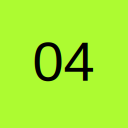# Multiple sheets using vlookups to return answer

• I have multiple sheets of data within my workbook that have the same column headers and I am trying to combine an If statement and nested vlookups to return a value if found. This is the latest formula I have tried, but it is still not working;

=IF(ISERROR(VLOOKUP(\$A5,'1-to-1 Match FAs'!\$A:\$AG,22,FALSE)),(VLOOKUP(\$A5,'OOMs by FA Totals <>'!\$A:\$AG,22,FALSE)))

Thanks
0411

• Re: Multiple sheets using vlookups to return answer

How many are the multiple sheets? and what Excel version are you using?

Where there is a will there are many ways. Finding one that works for you is the challenge!

• Re: Multiple sheets using vlookups to return answer

It's hard to help without seeing the structure of your data. Can you attach a sample workbook (with dummy data in the same structure) so we can help>

• Re: Multiple sheets using vlookups to return answer

Sorry I should have mentioned that to begin with - 2 sheets, Excel 2007

• Re: Multiple sheets using vlookups to return answer

Perhaps then:

=IFERROR(VLOOKUP(\$A5,'1-to-1 Match FAs'!\$A:\$AG,22,FALSE),VLOOKUP(\$A5,'OOMs by FA Totals <>'!\$A:\$AG,22,FALSE))

or

=IFERROR(VLOOKUP(\$A5,'1-to-1 Match FAs'!\$A:\$AG,22,FALSE),IFERROR(VLOOKUP(\$A5,'OOMs by FA Totals <>'!\$A:\$AG,22,FALSE),"")) to return a blank if none match.

Where there is a will there are many ways. Finding one that works for you is the challenge!

• Re: Multiple sheets using vlookups to return answer

forum.ozgrid.com/index.php?attachment/47894/

I have attached a dummy workbook - the first tab is where my formula's are, the 2nd & 3rd tabs are what I am using my vlookups against. Thanks for your help

0411

## Files

• Re: Multiple sheets using vlookups to return answer

The 2nd formula worked perfectly! Thanks so much
0411

## Participate now!

Don’t have an account yet? Register yourself now and be a part of our community!